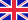•# First Grade Math Workbook

Autor: Isabella Hart

Get your kid ready for school with Addition & Subtraction Workbook!¿ ¿

Practice is included for single digit, double-digit addition and subtraction, counting, and more. Our books are packed with plenty of fun activities that teach a variety of essential... Viac o knihe

Na objednávku, dodanie 2-4 týždne

7.31 €

bežná cena: 8.60 €

## O knihe

Get your kid ready for school with Addition & Subtraction Workbook!¿ ¿

Practice is included for single digit, double-digit addition and subtraction, counting, and more. Our books are packed with plenty of fun activities that teach a variety of essential school skills.

Cute and inspiring themes. All the activities involve a variety of magical creatures and animals to keep your child engaged.

Why You Will Love This Book:

¿¿ Over 50 addition & subtraction worksheets

¿¿ Perfectly sized at 8.5" x 11"

¿¿ Carefully designed for 1st Grade

¿¿ Practice addition and subtraction in a fun and easy way

Kws: first grade workbook, 1st grade math, first grade math, common core math grade 1, math 1st grade, 1st grade math workbook, math for 1st grade, 1st grade math book, easy math for kids, grade 1 math, math first grade, 1st grade workbooks math, first grade subtraction workbook, 1 grade math workbook, 1 st grade math, math for 1st graders, children math workbooks, mathematics grade 1, 1st grade super math success, 1st grade workbook math, first grade addition, 1st grade math worksheets, math worksheets grade 1, 1st grade math workbooks, math workbooks grade 1, first grade math workbook, addition and subtraction workbook, math grade 1, math workbook grade 1, addition and subtraction workbook grade 1

• Vydavateľstvo: Isabella Hart
• Rok vydania: 2020
• Formát: Paperback
• Rozmer: 280 x 216 mm
• Jazyk: Anglický jazyk
• ISBN: 9781715891602

Generuje redakčný systém BUXUS CMS spoločnosti ui42.